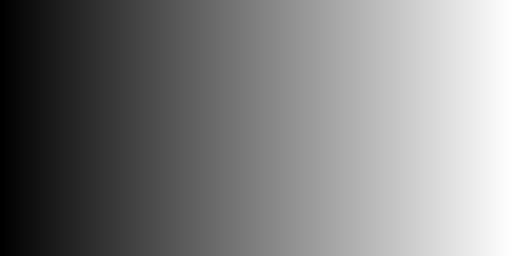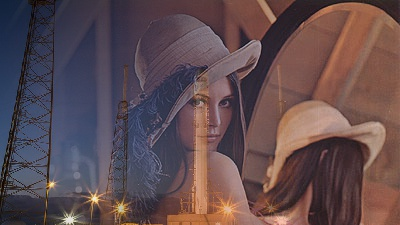# Composite two images according to a mask image with Python, Pillow

Posted: 2019-05-14 / Tags: Python, Pillow, Image Processing

In the `Image` module of the image processing library Pillow (PIL) of Python, `composite()` for compositing two images according to a mask image is provided.

• Parameters of `Image.composite()`
• Sample code of `Image.composite()`
• Composite the whole area at a uniform rate
• Create mask image by drawing
• Use existing image as mask image

Please refer to the following article for the installation and basic usage of Pillow (PIL).

Note that `composite()` is a function to composite two images of the same size. Use the `paste()` to composite images of different sizes. `paste()` allows you to mask a small image and paste it anywhere on the large image.

Image composition is possible with OpenCV and NumPy instead of Pillow. See the article below.

## Parameters of Image.composite()

There are three parameters for `composite()`. All three must be `Image` objects, all of the same size.

### image1, image2

Two images to composite.

`mode` must be one of the following three types.

• `1`: 1 bit image (binary image)
• `L`: 8-bit grayscale image
• `RGBA`: Image with alpha channel

`image1` and `image2` are alpha-blended according to the value of `mask`.

``````# For 1bit

# For 8bit
result = mask / 255 * image1 + (1 - mask / 255 ) * image2
``````

## Sample code of Image.composite()

Import `Image` from `PIL` and load images.

`ImageDraw` and `ImageFilter` are used when drawing a figure and creating a mask image. When reading an image file and using it as a mask image, they may be omitted.

``````from PIL import Image, ImageDraw, ImageFilter

im1 = Image.open('data/src/lena.jpg')
im2 = Image.open('data/src/rocket.jpg').resize(im1.size)
``````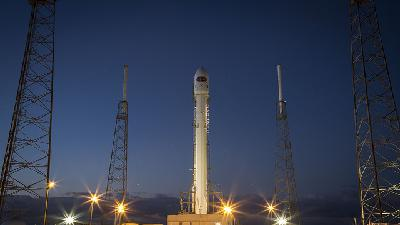This time, the second image is reduced by `resize()` to match the size. If you cut out part of the image and adjust the size, use `crop()`. See the following article.

### Composite the whole area at a uniform rate

When a solid image is used as a mask image, the entire image is composited at a uniform ratio.

As an example, create a solid image with a value of 128 with `Image.new()` and use it as a mask image.

``````mask = Image.new("L", im1.size, 128)
# im = Image.blend(im1, im2, 0.5)
``````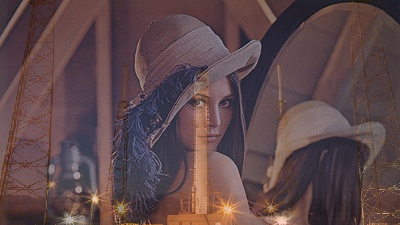`blend()` method can also be used if you want to composite the entire surface at a uniform ratio. Specify a constant of `0.0` to `1.0` as the parameter `alpha` instead of `mask`.

### Create mask image by drawing

If you want to mask and composite with simple shape such as circle and rectangle, drawing with `ImageDraw` module is convenient. For details on drawing, see the following article. You can also draw polygons.

Draw a white circle on a black background to create a mask image.

``````mask = Image.new("L", im1.size, 0)
draw.ellipse((140, 50, 260, 170), fill=255)
``````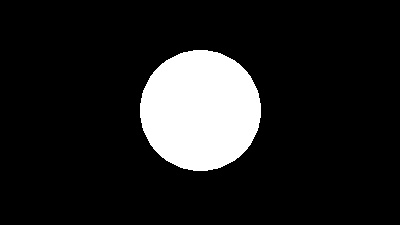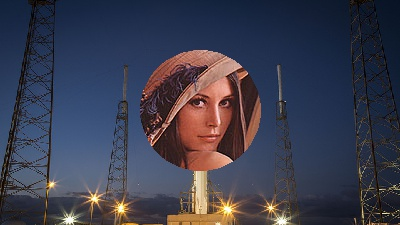The boundaries can be composited smoothly by blurring the mask image with `ImageFilter`.

``````mask_blur = mask.filter(ImageFilter.GaussianBlur(10))
``````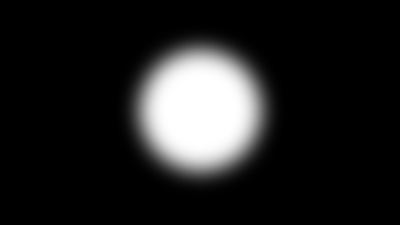### Use existing image as mask image

An existing image can be read and used as a mask image. It makes possible to composite in complex shape.

Try using a black and white horse-shaped image (scikit-image sample: skimage.data.horse()).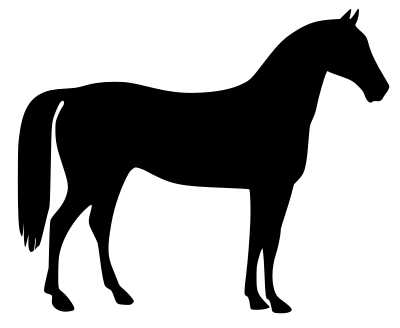After the image is read by `open()`, it is adjusted to the size of the pasted image by `resize()`, and the mode is converted to `'L'` (gray scale) by `convert()`.

``````mask = Image.open('data/src/horse.png').convert('L').resize(im1.size)
``````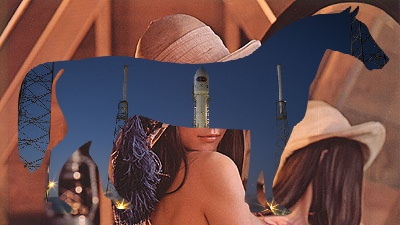If you want to reverse the black and white of the mask image, please refer to the following article.

As another example, it is composited so as to gradually change spatially using the gradation image. The gradation image was generated using NumPy.

``````mask = Image.open('data/src/gradation_h.jpg').convert('L').resize(im1.size)